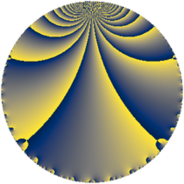# Properties

 Label 143.4.oLevel $143$ Weight $4$ Character orbit 143.o Rep. character $\chi_{143}(32,\cdot)$ Character field $\Q(\zeta_{12})$ Dimension $160$ Newform subspaces $1$ Sturm bound $56$ Trace bound $0$

# Related objects

## Defining parameters

 Level: $$N$$ $$=$$ $$143 = 11 \cdot 13$$ Weight: $$k$$ $$=$$ $$4$$ Character orbit: $$[\chi]$$ $$=$$ 143.o (of order $$12$$ and degree $$4$$) Character conductor: $$\operatorname{cond}(\chi)$$ $$=$$ $$143$$ Character field: $$\Q(\zeta_{12})$$ Newform subspaces: $$1$$ Sturm bound: $$56$$ Trace bound: $$0$$

## Dimensions

The following table gives the dimensions of various subspaces of $$M_{4}(143, [\chi])$$.

Total New Old
Modular forms 176 176 0
Cusp forms 160 160 0
Eisenstein series 16 16 0

## Trace form

 $$160 q - 4 q^{3} - 12 q^{4} - 8 q^{5} - 652 q^{9} + O(q^{10})$$ $$160 q - 4 q^{3} - 12 q^{4} - 8 q^{5} - 652 q^{9} - 80 q^{11} - 64 q^{14} - 76 q^{15} + 940 q^{16} + 68 q^{20} + 28 q^{22} - 240 q^{23} + 496 q^{26} + 824 q^{27} - 280 q^{31} - 266 q^{33} + 2212 q^{34} - 2760 q^{36} + 328 q^{37} + 1164 q^{42} + 104 q^{44} + 896 q^{45} + 4 q^{47} + 2080 q^{48} - 12 q^{49} - 6528 q^{53} + 682 q^{55} - 1356 q^{56} + 1096 q^{58} - 1392 q^{59} + 4 q^{60} - 1880 q^{66} + 304 q^{67} - 12 q^{69} - 1932 q^{70} - 5076 q^{71} + 8832 q^{75} - 10876 q^{78} + 4588 q^{80} - 4624 q^{81} - 7716 q^{82} + 5608 q^{86} + 10152 q^{88} + 7268 q^{89} - 1008 q^{91} + 8120 q^{92} + 2740 q^{93} + 2728 q^{97} - 6996 q^{99} + O(q^{100})$$

## Decomposition of $$S_{4}^{\mathrm{new}}(143, [\chi])$$ into newform subspaces

Label Dim $A$ Field CM Traces $q$-expansion
$a_{2}$ $a_{3}$ $a_{5}$ $a_{7}$
143.4.o.a $160$ $8.437$ None $$0$$ $$-4$$ $$-8$$ $$0$$